The easiest word problems

1. Three cats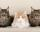If three cats eat three mice in three minutes, after which time 260 cats eat 260 mice?
2. Chocolate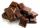Leslie bought 8 same chocolates for 16 Eur. How many euros will he pay for 25 chocolates?
3. DivisibilityIs the number 761082 exactly divisible by 9? (the result is the integer and/or remainder is zero)
4. Inflation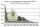What is better for people (employees)? ?
5. Brick weightThe brick weighs 2 kg and a half bricks. How much does one brick weigh?
6. One halfOne half of ? is: ?
7. Family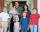The parents had 7 daughters. Each of them had one brother. How many members family had?
8. Cyclist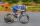A cyclist passes 88 km in 4 hours. How many kilometers he pass in 8 hours?
9. Orchard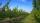3 trees in 3 lines grows in the orchard. How many trees are in the orchard?
10. Balls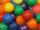I have 19 balls and my friend has 27. About how many has my friend more balls?
11. Ton's teeth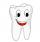Calculate at what age homeless Anton will toothless. At age 29 has 26 teeth and each year next one tooth broke.
12. Holidays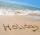Calculate how many hours take holidays, if take 5 weeks.
13. 7 heroes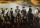9 heroes galloping on 9 horses behind. How many ways can sort them behind?
14. Proof PTCan you easy prove Pythagoras theorem using Euclidean theorems? If so, do it.
15. Vans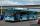In how many ways can 9 shuttle vans line up at the airport?
16. Reverse Pythagorean theoremGiven are lengths of the sides of the triangles. Decide which one is rectangular: Δ ABC: 77 dm, 85 dm, 36 dm ? Δ DEF: 55 dm, 82 dm, 61 dm ? Δ GHI: 24 mm, 25 mm, 7 mm ? Δ JKL: 32 dm, 51 dm, 82 dm ? Δ MNO: 51 dm, 45 dm, 24 dm ?
17. Piggy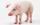A man bought a pig for € 60 then sold it for € 75. Then again bought for € 90 and sold it for € 100. How many € earned?
18. RootThe root of the equation ? is: ?
19. Is right?Is triangle with sides 51, 56 and 77 right triangle?
20. Weeks38 weeks is equal to how many days?

Do you have an interesting mathematical word problem that you can't solve it? Enter it, and we can try to solve it.

To this e-mail address, we will reply solution; solved examples are also published here. Please enter e-mail correctly and check whether you don't have a full mailbox.